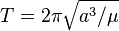# Possibility of multiple moons in Earth-Moon system

I am interested in theoretical (what if) possibility of our Earth having more than one moon,
I wonder if they can be arranged at the same orbital resonance as the Galilean moons 1:2:4

If yes, would such system be stable?
If yes, should our Moon be the first, second or third satellite out of the three for the system to be most stable?

Assuming such system is possible and stable,
I have made some calculations based on the assumption that our Moon is second (Moon2), and using the Kepler's Third Law, for calculating the distance of all the moons as function of the orbital period T (see Orbital Period wiki):Moon1 distance: (((27.3*24*60*60)^2*398600)/(16*3.14^2))^(1/3) = 241,000 Km

Moon2 distance: (((27.3*24*60*60)^2*398600)/(4*3.14^2))^(1/3) = 383,000 Km

Moon3 distance: (((27.3*24*60*60)^2*398600)/(1*3.14^2))^(1/3) = 608,000 Km

is this correct way to do this, or am i missing something, and this is not the way to calculate this distances?
If no, please point me to correct info,
Thanks,

Bandersnatch
is this correct way to do this, or am i missing something, and this is not the way to calculate this distances?
Looks fine. Although you didn't have to use all those numbers. It's much easier to just use ratios:
In units of Lunar month and Lunar orbital radius, you can just write:
##a=\sqrt{T^2}##
which will net you the ratio of Lunar orbital radius. E.g., for ##T=2## you get a=1.59 (of the radius), which is about 608 Mm.

I am interested in theoretical (what if) possibility of our Earth having more than one moon
This paper:
Formation of Regular Satellites from Ancient Massive Rings in the Solar System
suggests it being unlikely for Earth to form a multiple satellite system. At least as a result of accretion from ring material.

The specific question of the most stable configuration I won't be able to answer, sorry. Naively, I'd think if it works for Jupiter...

Naively, I'd think if it works for Jupiter...
...it is not allowed for ox.

But Moon is pretty far out and therefore strongly perturbed by Sun. Satellites outside Moon would therefore be expected to be in trouble.

Thanks Bandersnatch, your representation is much nicer indeed.

the paper is very interesting, it will take me some time to swallow it.

Moon is pretty far out and therefore strongly perturbed by Sun. Satellites outside Moon would therefore be expected to be in trouble.
This is something that I was afraid of, but without some simulation or numerical calculation I can't know for sure.

Also, for the 1:2:4 orbital resonance rule, what is the maximum possible masses of the satellites that satisfy such rule? can it be that our Moon is too massive to allow for other satellites to exist even if they try to follow this orbital resonance?

mfb
Mentor
More moons are problematic. Our moon is very massive compared to earth, so it will disturb other orbits significantly.
The outer border for moons is given by the hill sphere. I don't think you can fit a stable orbit between moon and the outer region of stability for earth satellites.

Stable low earth orbits (far away from the moon) are easy. Resonances I don't know. The orbit of moon is not circular, and changes its orientation over time due to perturbations from the sun. It would be tricky to fit a resonant orbit in that I think.

More moons are problematic. Our moon is very massive compared to earth, so it will disturb other orbits significantly.
Charon is even more massive, but is resonant with Nix and Hydra. Yet these are from 1:4, not closer in or inside Charon orbit.

BobG